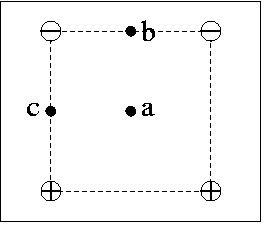# Problem: Four point charges of equal magnitude are arranged at the corners of a square as shown in the figure. Positive charges are indicated by "+" and negative charges are indicated by "-".What is the direction of the electric field at point b?A. To the left.B. To the rightC. Down (to the bottom of the page).D. The electric field is zero at this point.E. Up (to the top of the page).

###### FREE Expert Solution

The electric field is always directed from the positive charge to the negative charge.

85% (229 ratings)###### Problem Details

Four point charges of equal magnitude are arranged at the corners of a square as shown in the figure. Positive charges are indicated by "+" and negative charges are indicated by "-".What is the direction of the electric field at point b?

A. To the left.

B. To the right

C. Down (to the bottom of the page).

D. The electric field is zero at this point.

E. Up (to the top of the page).

Frequently Asked Questions

What scientific concept do you need to know in order to solve this problem?

Our tutors have indicated that to solve this problem you will need to apply the Electric Field concept. You can view video lessons to learn Electric Field. Or if you need more Electric Field practice, you can also practice Electric Field practice problems.

What professor is this problem relevant for?

Based on our data, we think this problem is relevant for Professor Hill's class at FSU.